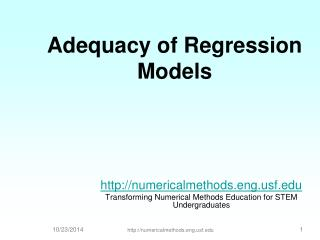# Adequacy of Regression Models - PowerPoint PPT PresentationDownload PresentationDownload Presentation- - - - - - - - - - - - - - - - - - - - - - - - - - - E N D - - - - - - - - - - - - - - - - - - - - - - - - - - -
##### Presentation Transcript

1. Adequacy of Regression Models http://numericalmethods.eng.usf.edu Transforming Numerical Methods Education for STEM Undergraduates 10/23/2014 http://numericalmethods.eng.usf.edu 1

2. Data

3. Is this adequate? Straight Line Model

4. Quality of Fitted Data • Does the model describe the data adequately? • How well does the model predict the response variable predictably?

5. Linear Regression Models • Limit our discussion to adequacy of straight-line regression models

6. Four checks • Plot the data and the model. • Find standard error of estimate. • Calculate the coefficient of determination. • Check if the model meets the assumption of random errors.

7. END

8. 1. Plot the data and the model

9. Data and model

10. END

11. 2. Find the standard error of estimate

12. Standard error of estimate

13. Standard Error of Estimate

14. Standard Error of Estimate

15. Standard Error of Estimate

16. Scaled Residuals 95% of the scaled residuals need to be in [-2,2]

17. Scaled Residuals

18. END

19. 3. Find the coefficient of determination

20. Coefficient of determination

21. y x Sum of square of residuals between data and mean

22. y x Sum of square of residuals between observed and predicted

23. Limits of Coefficient of Determination

24. Calculation of St

25. Calculation of Sr

26. Coefficient of determination

27. Caution in use of r2 • Increase in spread of regressor variable (x) in y vs. x increases r2 • Large regression slope artificially yields high r2 • Large r2 does not measure appropriateness of the linear model • Large r2 does not imply regression model will predict accurately

29. Final Exam Grade vs Pre-Req GPA

30. END

31. 4. Model meets assumption of random errors

32. Model meets assumption of random errors • Residuals are negative as well as positive • Variation of residuals as a function of the independent variable is random • Residuals follow a normal distribution • There is no autocorrelation between the data points.

33. Therm exp coeff vs temperature

34. Data and model

35. Plot of Residuals

36. Histograms of Residuals

37. Check for Autocorrelation • Find the number of times, qthe sign of the residual changes for the n data points. • If (n-1)/2-√(n-1) ≤q≤ (n-1)/2+√(n-1), you most likely do not have an autocorrelation.

38. Is there autocorrelation?

39. y vs x fit and residuals n=40 (n-1)/2-√(n-1) ≤p≤ (n-1)/2+√(n-1) Is 13.3≤21≤ 25.7? Yes!

40. y vs x fit and residuals n=40 (n-1)/2-√(n-1) ≤p≤ (n-1)/2+√(n-1) Is 13.3≤2≤ 25.7? No!

41. END

42. What polynomial model to choose if one needs to be chosen?

43. First Order of Polynomial

44. Second Order Polynomial

45. Which model to choose?

46. Optimum Polynomial

47. THE END

48. Effect of an Outlier

49. Effect of Outlier